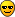# From covariance matrix to chi2 distribution

Dear Experts,

Testing how accurate the covariance matrix from Minuit is, I found that a factor 2 is missing on the matrix, but I cannot figure out why. Here what I’m doing:

1. I define a FCN as in . A log-likelihood function with no 2 factor on top of it.
2. I set “gMinuit->mnexcm(“SET ERR”,arglist,1,ierflg)”, where arglist=0.5; As it should be I believe.
3. I fit 8 parameters, but I’m mostly interested in 6 of them. So I extract the covariance matrix, and I take the 6x6 components relative to the parameters I want to study.
4. If I translate the 6D covariance matrix into a 6D ellipsoid, I can check how many time the true value of the parameters is inside such ellipsoid.
5. In particular, I want to solve the equation:
Vector(p1,p2,p3…) * Cov_matrix-1 * Vector(p1,p2,p3…) = delta_chi2
where Cov_matrix-1 is the inverse of the cov matrix, and Vector(p1,p2,p3…) is the 6D vector of the difference between the true value and the fitted value of each parameter.Solving the equation for each fit I perform (250 fits), I should find 250 values, that should follow the chi2 distribution for 6 ndof.

-> What I notice that I miss exactly a factor 2 in the covariance matrix in order to get a perfect chi2 distribution for 6 ndof. Do you know where this factor 2 could be missing?
I tried using MINOS, HESSE, strategy 1 and 2. All results are consistent (and I think this is good from one side).

Thanks a lot,
Luca


static const double cgaus = 0.5 * log( 2.*M_PI );
return -((-pow(residual - center, 2) *0.5 / sigma / sigma) - cgaus - log(sigma));
 seal.web.cern.ch/seal/documents/ … nerror.pdf

Dear Experts,

I can confirm that playing with “SET ERR” is not helping.
Also using MINOS, HESSE, strategy 1 and 2 give similar results.
Any help in understanding why the size of the covariance matrix is missing a factor 2 would be appreciated.

Thanks a lot,
Luca